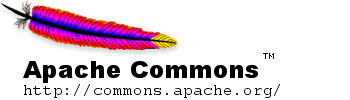## 3 Linear Algebra

### 3.1 Overview

Linear algebra support in commons-math provides operations on real matrices (both dense and sparse matrices are supported) and vectors. It features basic operations (addition, subtraction ...) and decomposition algorithms that can be used to solve linear systems either in exact sense and in least squares sense.

### 3.2 Real matrices

The RealMatrix interface represents a matrix with real numbers as entries. The following basic matrix operations are supported:

• Matrix addition, subtraction, multiplication
• Scalar addition and multiplication
• transpose
• Norm and Trace
• Operation on a vector

Example:

// Create a real matrix with two rows and three columns, using a factory
// method that selects the implementation class for us.
double[][] matrixData = { {1d,2d,3d}, {2d,5d,3d}};
RealMatrix m = MatrixUtils.createRealMatrix(matrixData);

// One more with three rows, two columns, this time instantiating the
// RealMatrix implementation class directly.
double[][] matrixData2 = { {1d,2d}, {2d,5d}, {1d, 7d}};
RealMatrix n = new Array2DRowRealMatrix(matrixData2);

// Note: The constructor copies  the input double[][] array in both cases.

// Now multiply m by n
RealMatrix p = m.multiply(n);
System.out.println(p.getRowDimension());    // 2
System.out.println(p.getColumnDimension()); // 2

// Invert p, using LU decomposition
RealMatrix pInverse = new LUDecomposition(p).getSolver().getInverse();


The three main implementations of the interface are Array2DRowRealMatrix and BlockRealMatrix for dense matrices (the second one being more suited to dimensions above 50 or 100) and SparseRealMatrix for sparse matrices.

### 3.3 Real vectors

The RealVector interface represents a vector with real numbers as entries. The following basic matrix operations are supported:

• Vector addition, subtraction
• Element by element multiplication, division
• Scalar addition, subtraction, multiplication, division and power
• Mapping of mathematical functions (cos, sin ...)
• Dot product, outer product
• Distance and norm according to norms L1, L2 and Linf

The RealVectorFormat class handles input/output of vectors in a customizable textual format.

### 3.4 Solving linear systems

The solve() methods of the DecompositionSolver interface support solving linear systems of equations of the form AX=B, either in linear sense or in least square sense. A RealMatrix instance is used to represent the coefficient matrix of the system. Solving the system is a two phases process: first the coefficient matrix is decomposed in some way and then a solver built from the decomposition solves the system. This allows to compute the decomposition and build the solver only once if several systems have to be solved with the same coefficient matrix.

For example, to solve the linear system

           2x + 3y - 2z = 1
-x + 7y + 6x = -2
4x - 3y - 5z = 1

Start by decomposing the coefficient matrix A (in this case using LU decomposition) and build a solver
RealMatrix coefficients =
new Array2DRowRealMatrix(new double[][] { { 2, 3, -2 }, { -1, 7, 6 }, { 4, -3, -5 } },
false);
DecompositionSolver solver = new LUDecomposition(coefficients).getSolver();

Next create a RealVector array to represent the constant vector B and use solve(RealVector) to solve the system
RealVector constants = new ArrayRealVector(new double[] { 1, -2, 1 }, false);
RealVector solution = solver.solve(constants);

The solution vector will contain values for x (solution.getEntry(0)), y (solution.getEntry(1)), and z (solution.getEntry(2)) that solve the system.

Each type of decomposition has its specific semantics and constraints on the coefficient matrix as shown in the following table. For algorithms that solve AX=B in least squares sense the value returned for X is such that the residual AX-B has minimal norm. Least Square sense means a solver can be computed for an overdetermined system, (i.e. a system with more equations than unknowns, which corresponds to a tall A matrix with more rows than columns). If an exact solution exist (i.e. if for some X the residual AX-B is exactly 0), then this exact solution is also the solution in least square sense. This implies that algorithms suited for least squares problems can also be used to solve exact problems, but the reverse is not true. In any case, if the matrix is singular within the tolerance set at construction, an error will be triggered when the solve method will be called, both for algorithms that compute exact solutions and for algorithms that compute least square solutions.

 Decomposition algorithms Name coefficients matrix problem type LU square exact solution only Cholesky symmetric positive definite exact solution only QR any least squares solution eigen decomposition square exact solution only SVD any least squares solution

It is possible to use a simple array of double instead of a RealVector. In this case, the solution will be provided also as an array of double.

It is possible to solve multiple systems with the same coefficient matrix in one method call. To do this, create a matrix whose column vectors correspond to the constant vectors for the systems to be solved and use solve(RealMatrix), which returns a matrix with column vectors representing the solutions.

### 3.5 Eigenvalues/eigenvectors and singular values/singular vectors

Decomposition algorithms may be used for themselves and not only for linear system solving. This is of prime interest with eigen decomposition and singular value decomposition.

The getEigenvalue(), getEigenvalues(), getEigenVector(), getV(), getD() and getVT() methods of the EigenDecomposition interface support solving eigenproblems of the form AX = lambda X where lambda is a real scalar.

The getSingularValues(), getU(), getS() and getV() methods of the SingularValueDecomposition interface allow to solve singular values problems of the form AXi = lambda Yi where lambda is a real scalar, and where the Xi and Yi vectors form orthogonal bases of their respective vector spaces (which may have different dimensions).

### 3.6 Non-real fields (complex, fractions ...)

In addition to the real field, matrices and vectors using non-real field elements can be used. The fields already supported by the library are: